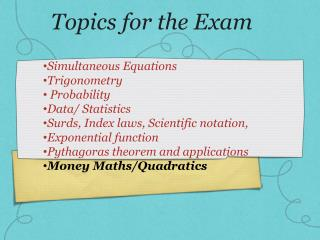DownloadDownload PresentationTopics for the Exam

# Topics for the Exam

Download Presentation## Topics for the Exam

- - - - - - - - - - - - - - - - - - - - - - - - - - - E N D - - - - - - - - - - - - - - - - - - - - - - - - - - -
##### Presentation Transcript

1. Topics for the Exam Simultaneous Equations Trigonometry Probability Data/ Statistics Surds, Index laws, Scientific notation, Exponential function Pythagoras theorem and applications Money Maths/Quadratics

2. All students must complete both sections Exam structure Section A • Simultaneous Equations • Trigonometry • Probability • Data/ Statistics • Surds, Index laws, Scientific notation, • Exponential function • Pythagoras theorem and applications • Section B • Money Maths • Or • Quadratics

3. Exam requirements • 1 side of handwritten A4 notes (cheat sheet) • Pen, pencil (for drawing a graph), eraser • Calculator – phones not allowed!

4. Pythagoras Theorem • The Pythagorean Theorem consists of the following: The sum of the • squares of two legs of a right triangle is equal to the hypotenuse • squared. 2 2 2 a + b = c

5. Baseball Diamond • How far does the second baseman have to throw the ball in order to get the runner out before he slides into the home plate?

6. Measurement of TV • Television sets are generally measured diagonally, thus classifying them as 13 inches, 27 inches, 36 inches, and so forth. • Suppose we want to purchase an entertainment center, but it only holds enough room in it’s cubicle for a 27 inch TV set. • We initially know that the length of our TV is 15 inches, and the height of our TV is 12 inches. • Will our TV be able to fit into the cubicle?

7. Pythagoras’ Theorem in 3D • A) Find the length AC • B) Find the length AD

8. Pythagoras’ Theorem in 3D • A) Find the length AC • B) Find the length AD • AC = √(52 + 52) = √50 • AD = √(52 + (√50) 2) • = √(25 + 50) = √75

9. Pythagoras’ Theorem in 3D For the square pyramid shown, calculate: • The length AC • The height EF AC = √(252 + 252) = √1250 = 35.35 262 = EF2 + AF2 EF = √(262 – 17.682) = 19.065

10. Pythagoras in 3 dimensions Find the length from A to D 4 First find the distance A to C…